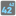• # Check problem? - Elementary / "Index Power" Task.

## Question related to missionIndex Power

### The task to be performed is as follows.

You are given an array with positive numbers and a number N. You should find the N-th power of the element in the array with the index N. If N is outside of the array, then return -1. Don't forget that the first element has the index 0.

Let's look at a few examples: - array = [1, 2, 3, 4] and N = 2, then the result is 32 == 9; - array = [1, 2, 3] and N = 3, but N is outside of the array, so the result is -1.

Example :

```indexPower([1, 2, 3, 4], 2) == 9
indexPower([1, 3, 10, 100], 3) == 1000000
indexPower([0, 1], 0) == 1
indexPower([1, 2], 3) == -1
```

The expected results are

```9
1000000
1
-1
```

I have achieved those results, I have checked it in the chrome console and also in the "run" of the site console, however it does not pass the check when arriving at the last calculation, which is this:

```indexPower([1, 2], 3)
```

In Chrome console and in the "run" of the exercise, I get -1, however the check informs me the following:

```Your result:null
Right result:-1
Fail:indexPower([96,92,94],3)
```

And that's where I'm stuck, I do not understand why the last calculation does not pass the check, am I doing something wrong? those figures that show me are not what I have in the code.

Just in case some charitable soul knows I'm making the mistake, could you tell me? I leave the code I did to analyze it. Thank you!

```"use strict";

function indexPower(array, n){

if (n < array.length) {

var valor = array[n] ** n;

} else if (n < 0 || n > array.length) {

valor = (n - n)-1;

}
return valor;
}

var assert = require('assert');

if (!global.is_checking) {
console.log('Example:')

console.log(indexPower([1, 2, 3, 4], 2));
console.log(indexPower([1, 3, 10, 100], 3));
console.log(indexPower([0, 1], 0));
console.log(indexPower([1, 2], 3));

assert.equal(indexPower([1, 2, 3, 4], 2), 9, "Square");
assert.equal(indexPower([1, 3, 10, 100], 3), 1000000, "Cube");
assert.equal(indexPower([0, 1], 0), 1, "Zero power");
assert.equal(indexPower([1, 2], 3), -1, "IndexError");
console.log("Coding complete? Click 'Check' to review your tests and earn cool rewards!");
}
```1